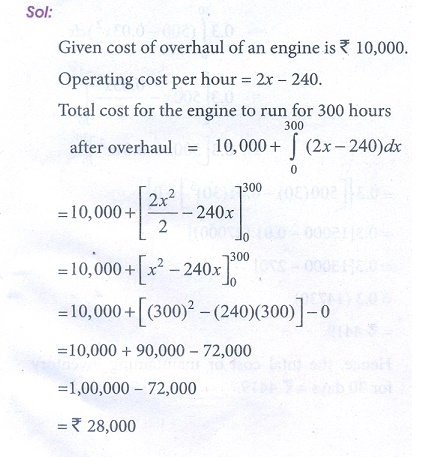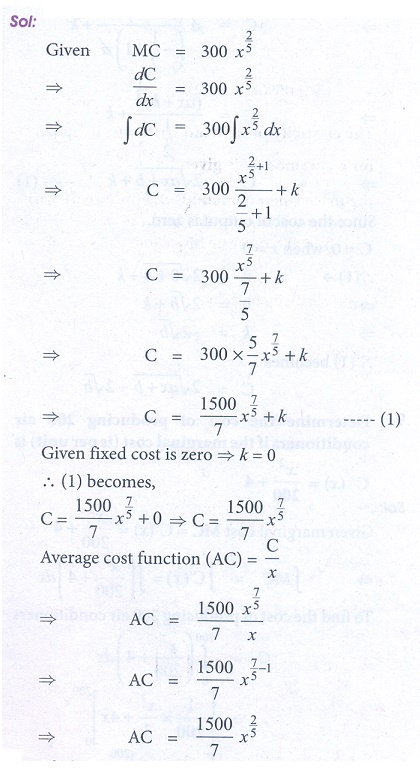Home | | Business Maths 12th Std | Exercise 3.2: Application of Integration in Economics and Commerce

# Exercise 3.2: Application of Integration in Economics and Commerce

Book back answers and solution for Exercise questions - Maths: Integral Calculus: Application of Integration in Economics and Commerce: Solved Problems with Answer, Solution, Formula

Exercise 3.2

1. The cost of over haul of an engine is Ōé╣10,000 The operating cost per hour is at the rate of 2x ŌłÆ 240 where the engine has run x km. Find out the total cost if the engine run for 300 hours after overhaul.2. Elasticity of a function Ey/ Ex is given by Ey/ Ex = ŌłÆ7x / (1 ŌłÆ 2x )( 2 + 3x ). Find the function when x = 2, y = 3/8 .3. The elasticity of demand with respect to price for a commodity is given by ( 4 ŌłÆ x) / x ,  where p is the price when demand is x. Find the demand function when price is 4 and the demand is 2. Also find the revenue function.4. A company receives a shipment of 500 scooters every 30 days. From experience it is known that the inventory on hand is related to the number of days x. Since the shipment, I ( x) = 500 ŌłÆ 0.03x2 , the daily holding cost per scooter is Ōé╣ 0.3. Determine the total cost for maintaining inventory for 30 days.5. An account fetches interest at the rate of 5% per annum compounded continuously An individual deposits Ōé╣1,000 each year in his account. How much will be in the account after 5 years. (e0.25 = 1.284) .6. The marginal cost function of a product is given by dC/dx = 100 ŌłÆ10x + 0.1x2 where x is the output. Obtain the total and the average cost function of the firm under the assumption, that its fixed cost is Ōé╣ 500.7. The marginal cost function is MC = 300 x2/5 and fixed cost is zero. Find out the total cost and average cost functions.8. If the marginal cost function of x units of output is a/ ŌłÜ[ax + b] and if the cost of output is zero. Find the total cost as a function of x.9. Determine the cost of producing 200 air conditioners if the marginal cost (is per unit) is C ŌĆ▓ ( x) = x2/200 + 4 .10. The marginal revenue (in thousands of Rupees) functions for a particular commodity is 5 + 3 eŌłÆ0 .03 x where x denotes the number of units sold. Determine the total revenue from the sale of 100 units. (Given eŌłÆ3 = 0.05 approximately)11. If the marginal revenue function for a commodity is MR = 9 ŌłÆ 4x2 . Find the demand function.12. Given the marginal revenue function 4/( 2x + 3)2  ŌłÆ 1 , show that the average revenue function is P = 4/[6x + 9] ŌłÆ1.13.  A firmŌĆÖs marginal revenue function is MR = 20e ŌłÆx 10 (1ŌłÆ x/10). Find the corresponding demand function.14. The marginal cost of production of a firm is given by C ŌĆ▓(x= 5 + 0.13x , the marginal revenue is given by R ŌĆ▓(x= 18 and the fixed cost is Ōé╣ 120. Find the profit function.15. If the marginal revenue function is R ŌĆ▓ ( x)= 1500 ŌłÆ 4x ŌłÆ 3x2 . Find the revenue function and average revenue function.16. Find the revenue function and the demand function if the marginal revenue for x units is MR= 10 + 3x ŌłÆ x2 .17. The marginal cost function of a commodity is given by MC = 14000/ŌłÜ[7x + 4] and the fixed cost is Ōé╣18,000.Find the total cost and average cost.18. If the marginal cost (MC) of a production of the company is directly proportional to the number of units (x) produced, then find the total cost function, when the fixed cost is Ōé╣ 5,000 and the cost of producing 50 units is Ōé╣ 5,625.19. If MR = 20 ŌłÆ 5x + 3x2 , find total revenue function.20. If MR = 14 ŌłÆ 6x + 9x2 , find the demand function.Tags : Problem Questions with Answer, Solution , 12th Business Maths and Statistics : Chapter 3 : Integral Calculus - II
Study Material, Lecturing Notes, Assignment, Reference, Wiki description explanation, brief detail
12th Business Maths and Statistics : Chapter 3 : Integral Calculus - II : Exercise 3.2: Application of Integration in Economics and Commerce | Problem Questions with Answer, Solution